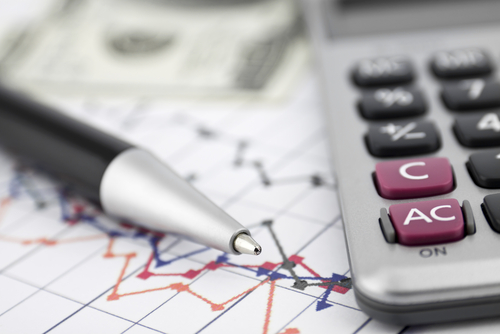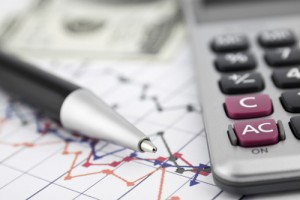## The What, Why and How of a Forex PIP Calculator

Sep 27 • Forex Calculator • 5634 Views • Comments Off on The What, Why and How of a Forex PIP CalculatorA pip is the acronym for “percentage in points”. This is Forex jargon for a unit of change for currency pairs in an exchange rate. As a general rule major currencies are valued up to 4 decimal places, except the Japanese Yen (JPY) which is valued at 2 decimal places. In other words the former refers to one unit in the fourth decimal point while the latter refers to one unit in the second decimal point.

It would take several, or most probably dozens of articles to fully discuss the importance of a pip. However for the purposes of this article, it is sufficient to understand that a pip is used to accurately determine the value at which a currency is traded every trading day as opposed to other currencies. Needless to say, an accurate Forex pip calculator is therefore a necessary tool for every trader. As such, this article will discuss the basics of the same.

What Is a Forex PIP Calculator?

A pip calculator is a tool used to determine different prices across different currencies and different lots. By way of analogy, think of a pip calculator as a cheat sheet of the multiplication table. The same may not be completely necessary, but is extremely helpful for beginners. This is because it ensures accurate computations to determine trading position and investment value. A pip calculator looks like a box with 2 selections. The first selection refers to the currency pair and the second selection refers to the position size. The result will be several figures that refer to what you will be trading for based on different currency prices.

Why Use a Forex PIP Calculator?

The simplest answer can be summed up in two words and these are “accuracy” and “convenience”. To be more specific, a pip calculator is necessary because Forex trading has very low margin requirements (you are talking about 4 decimal points as a general rule, remember). As such, you need a pip calculator to accurately determine the most advantageous currency pair and position so as to make the most amount of profit within your means. For example, a trader has to put up as little as \$200 to \$500 for a currency lot of \$100,000. Therefore, a few pips one way or the other means the difference between a substantial amount of profit and a disastrous loss.

How to Use a Forex PIP Calculator?

Here is where the discussion gets a bit tricky. Read the same once for familiarity, a second time for understanding and a third time for analysis. A Pip calculator is calculated using the base currency. This refers to the second currency in the currency pair. For example USD/EURO means that the base currency is EURO. Going back, this will then be multiplied via what is known as a lot size. As a general rule, a standard lot refers to 100,000 units. Of course some may be lower (nano lots) and some maybe higher.

In Closing

A Forex pip calculator is a useful tool. However, as in all tools, the same must be properly utilized in order to actually create the desired results. Of course you also need to take into consideration the nature of the foreign exchange market, which is volatile and liquid.